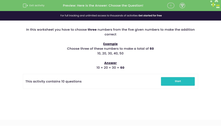# Here is the Answer: Choose the Question!

In this worksheet, students choose three numbers from a list of five numbers to make the addition correct.Key stage:  KS 2

Curriculum topic:   Number: Addition and Subtraction

Curriculum subtopic:   Solve Add/Subtract Problems to 1000

Difficulty level:#### Worksheet Overview

In this worksheet you have to choose three numbers from the five given numbers to make the addition correct

Example

Choose three of these numbers to make a total of 60

10, 20, 30, 40, 50